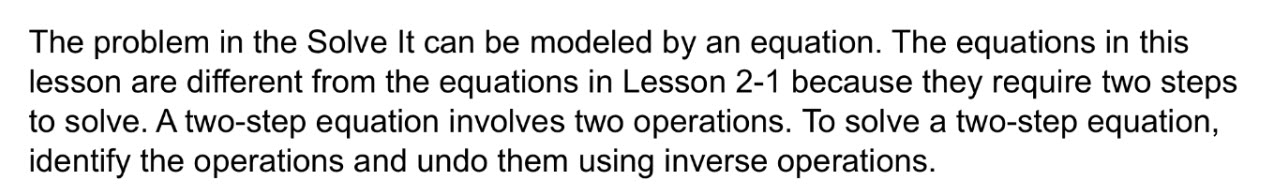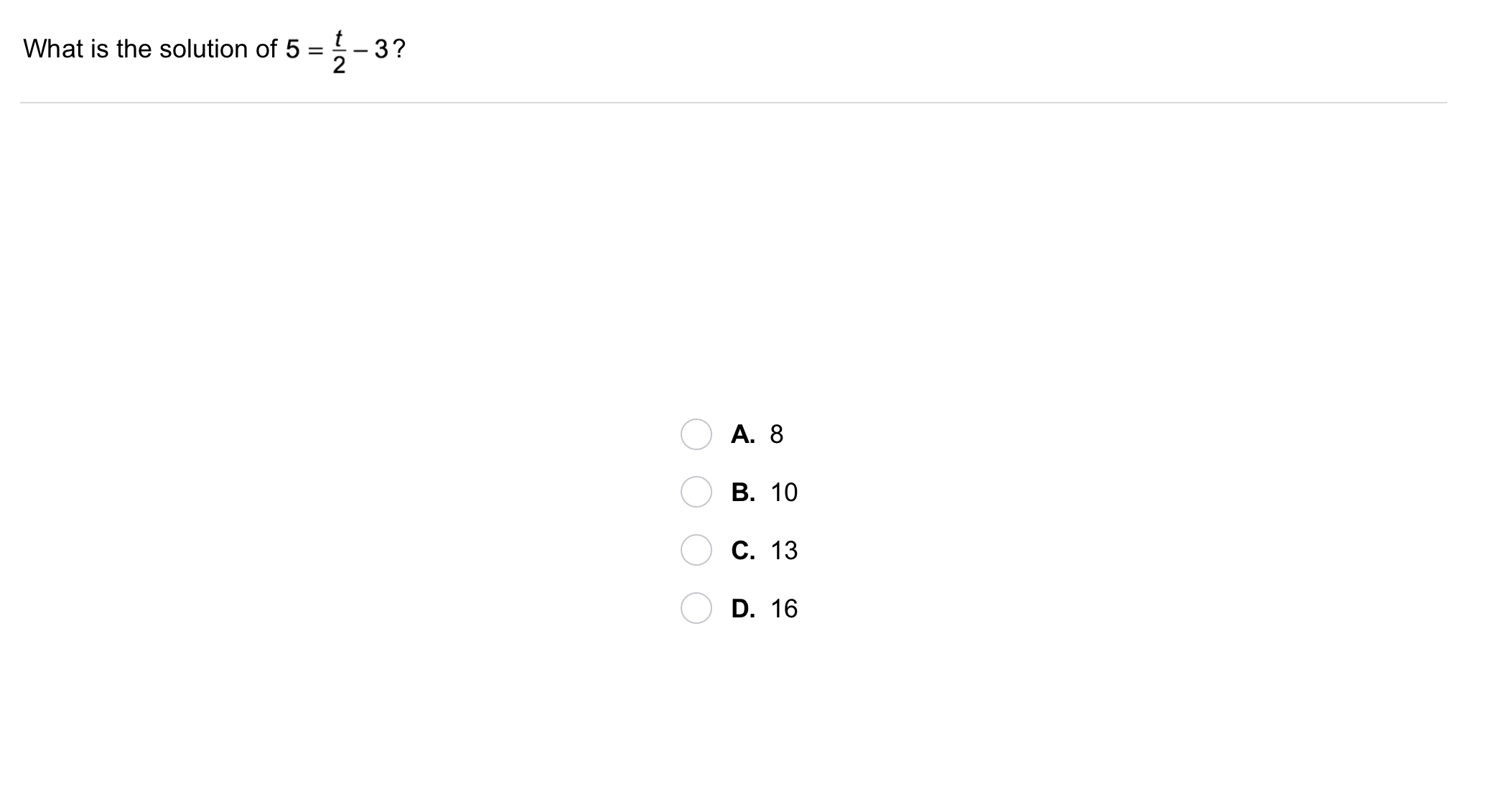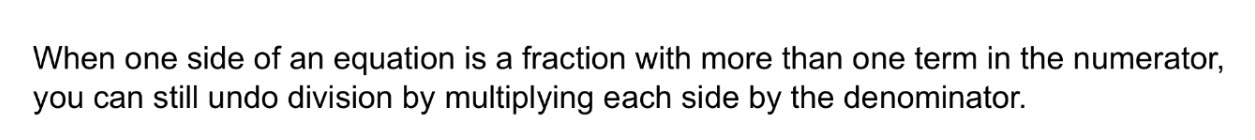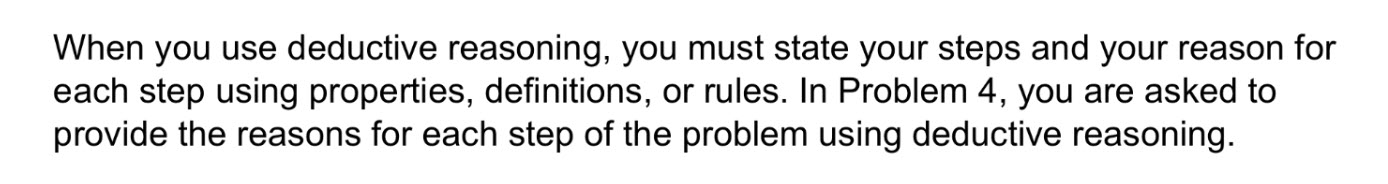Algebra 1 2-2 Guided Practice: Solving Two-Step Equations
starstarstarstarstarstarstarstarstarstar
by Matthew Richardson
| 12 Questions1
2
1
10
Solve It! The diagram shows the amount of money that each player starts with in a video game. To be fair, each player should have the same amount of money. If each chest contains the same amount of money, how much money must be in each chest? Enter only a number.Example of a two-step equation:3
4
5
d
3
10
Take Note: Provide an example of a two-step equation that includes mutliplication and addition.
4
10
Take Note: Recall that when we "do" math operations, we follow the order of operations, PEMDAS. Since solving equations is essentially "un-doing" operations, we reverse the order of operations. Order the following operations in the reverse order of PEMDAS.
1. parentheses & other grouping symbols
3. division/multiplication
4. exponents
5
10
Take Note: Summarize the process of solving a two-step equation.
Take Note: Just as PEMDAS is a helpful mnemonic device for remembering the order of operations, SADMEP can help you remember the order in which to "un-do" operations when solving equations.6
6
10
Problem 1 Got It?
A
B
C
D
7
10
Problem 2 Got It? Recall Problem 2:

Now, suppose you used one quarter of a sheet of paper for each ad and four full sheets for the title banner in Problem 2.

How many ads could you make?8
10
Enter only a number.
9
10
Problem 3 Got It? Write the right side of the equation as the difference of two fractions.11
11
10
Problem 4 Got It?
A
B
C
D12
12
10
Take Note: Summarize the mathematical content of this lesson. What topics, ideas, and vocabulary were introduced?